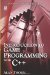7.3 Conditional Operators

7.3 Conditional Operators

In Chapter 2, conditional operators were examined alongside inequalities. C++ uses conditional operators to help programs make decisions about the data it encounters, as we shall see. To make decisions, a programmer needs to compare data. This is achieved using conditional operators, and these operators are as follows:

• = = Equal To

In C++, the = assignment operator is used to assign values to variables and constants. But the == equality operator is used to determine whether one value is equal to another.

• > Greater Than

Determines whether one quantity is larger than another.

• >= Greater Than or Equal To

Determines whether one quantity is greater than or equal to another.

• < Less Than

Checks to see whether one quantity is less than another.

• <= Less Than or Equal To

Checks whether one quantity is less than or equal to another.

• != Not Equal To

Tests whether one quantity is not equal to another.Introduction to Game Programming with C++ (Wordware Game Developers Library)
ISBN: 1598220322
EAN: 2147483647
Year: 2007
Pages: 225
Authors: Alan Thorn

Similar book on Amazon